# Bar Graphs

GCSEKS3Level 1-3AQACambridge iGCSEEdexcelEdexcel iGCSEOCRWJEC

## Bar Graphs

A bar graph (or bar chart) is a way of displaying data, typically data split into categories, using bars of different heights. Bar graphs are not the same thing as histograms, and you should make sure to leave gaps between the bars when drawing your bar graph so it doesn’t look like a histogram. You may also see vertical line charts. These do the same thing as bar charts, but a vertical line is used instead of a bar.

Understanding the following topics will help with bar graph questions:

Level 1-3GCSEKS3AQAEdexcelOCRWJECCambridge iGCSEEdexcel iGCSE

## How to Draw a Bar Graph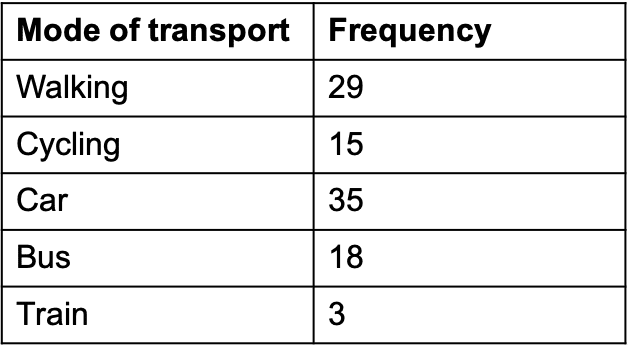$100$ students were asked what their primary mode of transport for getting to school was. The results of this survey are recorded in the table below. Construct a bar graph representing this information.

So, before drawing the bar graph, we have to consider what the requirements of our graph will be from the info in the table:

• There are $5$ categories, so it must be wide enough for $5$ bars,
• The highest frequency is $35$, meaning the scale on the $y$-axis must go at least at as high as $35$.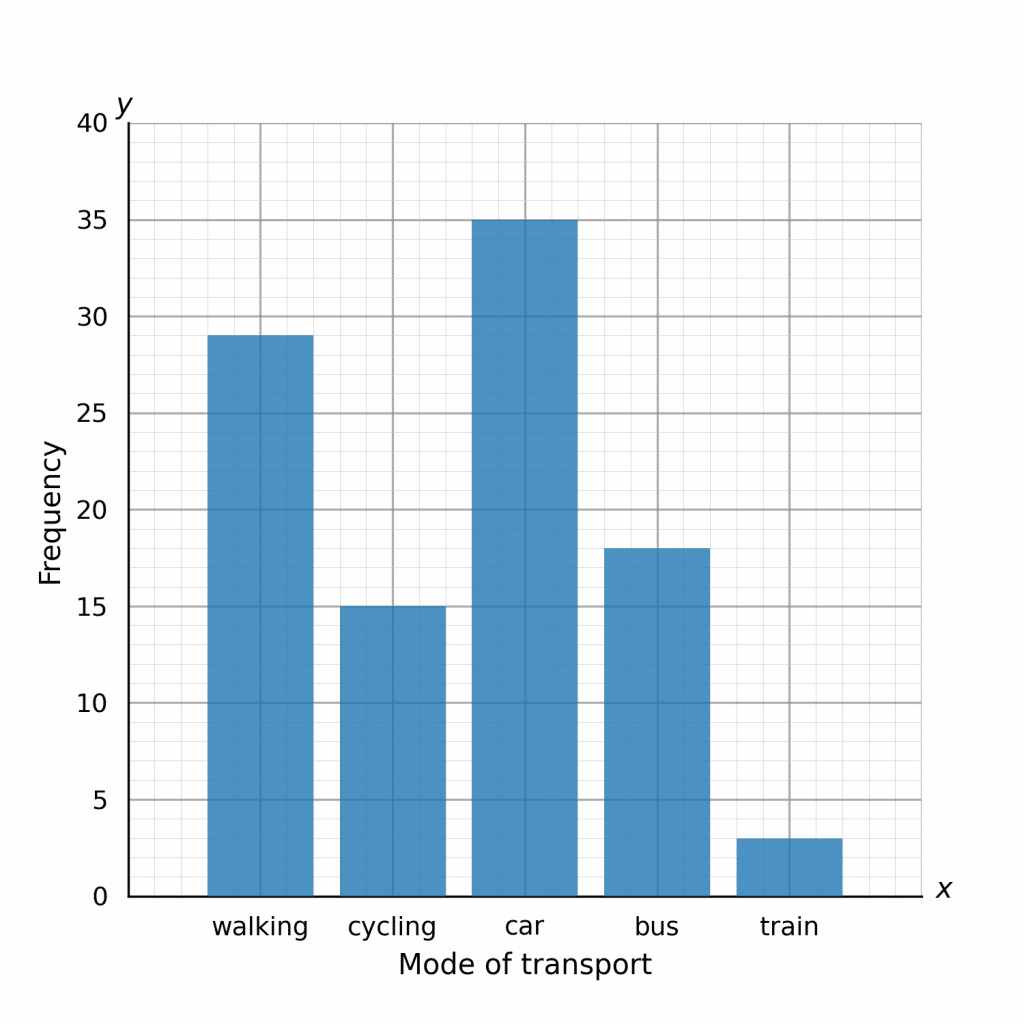Now, remembering to leave gaps between the bars and clearly labelling which bar represents which mode of transport, the resulting bar graph should look like the one shown.

As you can see, this bar graph displays the information in a clear and obvious way. The bar graph has the following features:

• The bars are very distinct, clearly labelled and have gaps.
• The scale on the $y$-axis is very readable: $1$ small square represents $1$ person.
• The scale chosen is appropriate to the data we were given.
• Both axes are labelled.
Level 1-3GCSEKS3AQAEdexcelOCRWJECCambridge iGCSEEdexcel iGCSE

## Understanding Bar Graphs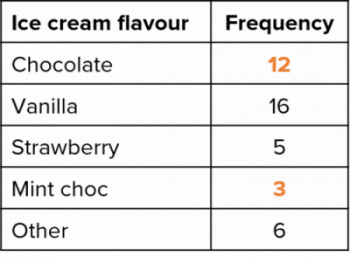A survey was conducted asking people about their favourite flavour of ice cream. The results of this survey are displayed on the bar chart.

a) Use the bar graph to fill in the gaps in the table.

To fill in the missing gaps in the table, we must read off the height of their corresponding bars from the bar chart.

We can see that each little square is worth $0.6$, and so the height of the ‘chocolate’ bar is $12$ and the height of the ‘mint choc chip’ bar is $3$.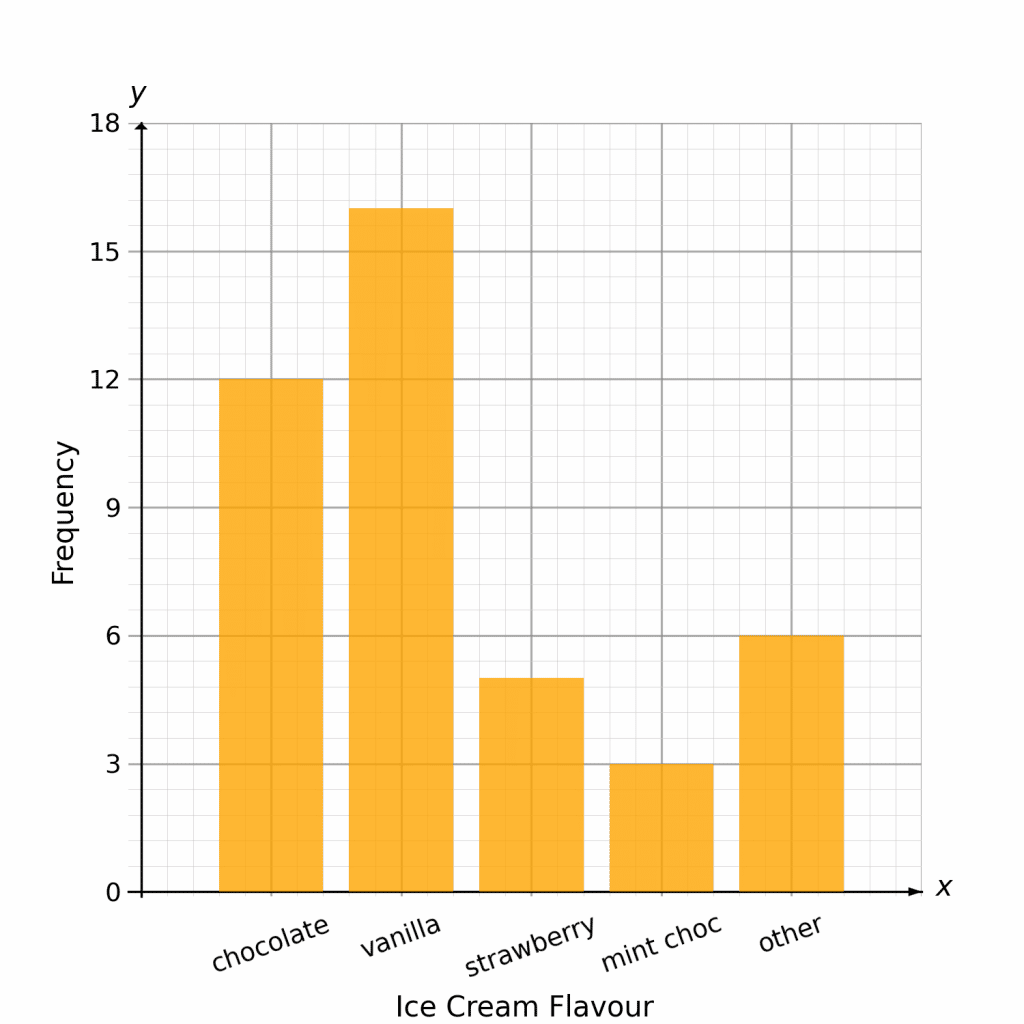b) Calculate the percentage of people surveyed whose favourite flavour is strawberry.

From the table, we can clearly see that $5$ people chose strawberry as their favourite. To find out what this is as a percentage, we need to work out how many people there were in total.

$\text{Total }=12+16+5+3+6=42$

Therefore, the percentage of people who chose strawberry is

$\dfrac{5}{42}\times 100=11.9\%\text{ (1 dp)}$

Level 1-3GCSEKS3AQAEdexcelOCRWJECCambridge iGCSEEdexcel iGCSE
Level 1-3GCSEKS3AQAEdexcelOCRWJECCambridge iGCSEEdexcel iGCSE

## Comparing Data Using Bar Graphs

If we want to compare two sets of data within the same context we can either plot both bar charts on the same graph or plot a composite bar chart.

Side by Side

The following bar chart shows the favourite school subjects of pupils in class $10\text{A}$ and $10\text{B}$.

We are then able to draw conclusions from the bar chart, e.g. English is more popular in class $10\text{B}$.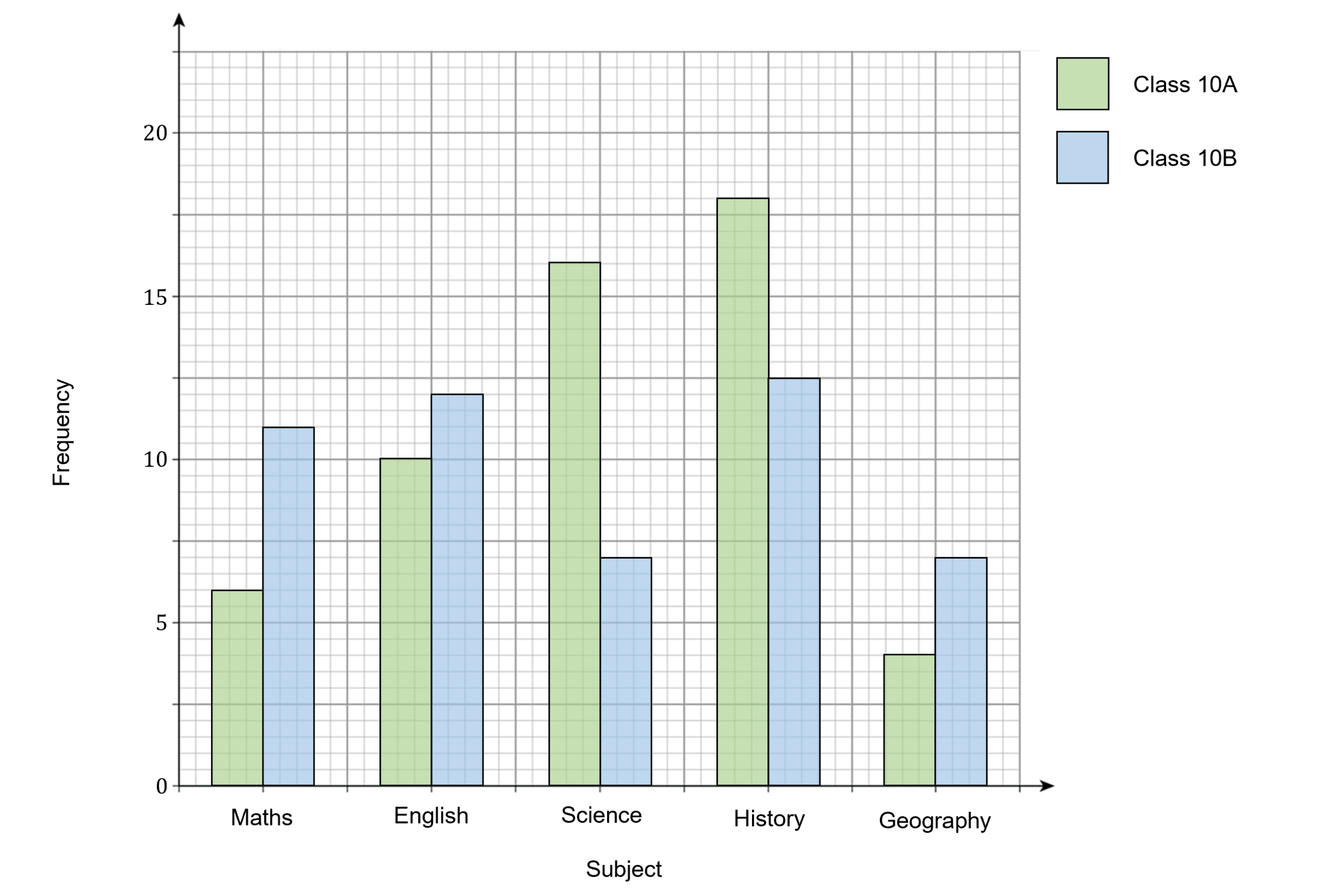Composite Bar Charts

Composite bar charts are another way of displaying more than one data set within the same context. Instead of the bars being side by side, they are stacked on top of each other.

For example, the bar chart below shows the number of sales at a clothes shop per day for both jeans and t-shirts.

We can see that on Monday there were $\textcolor{#10a6f3}{21}$ t-shirts sold and $\textcolor{#10a6f3}{8}$ pairs of jeans sold.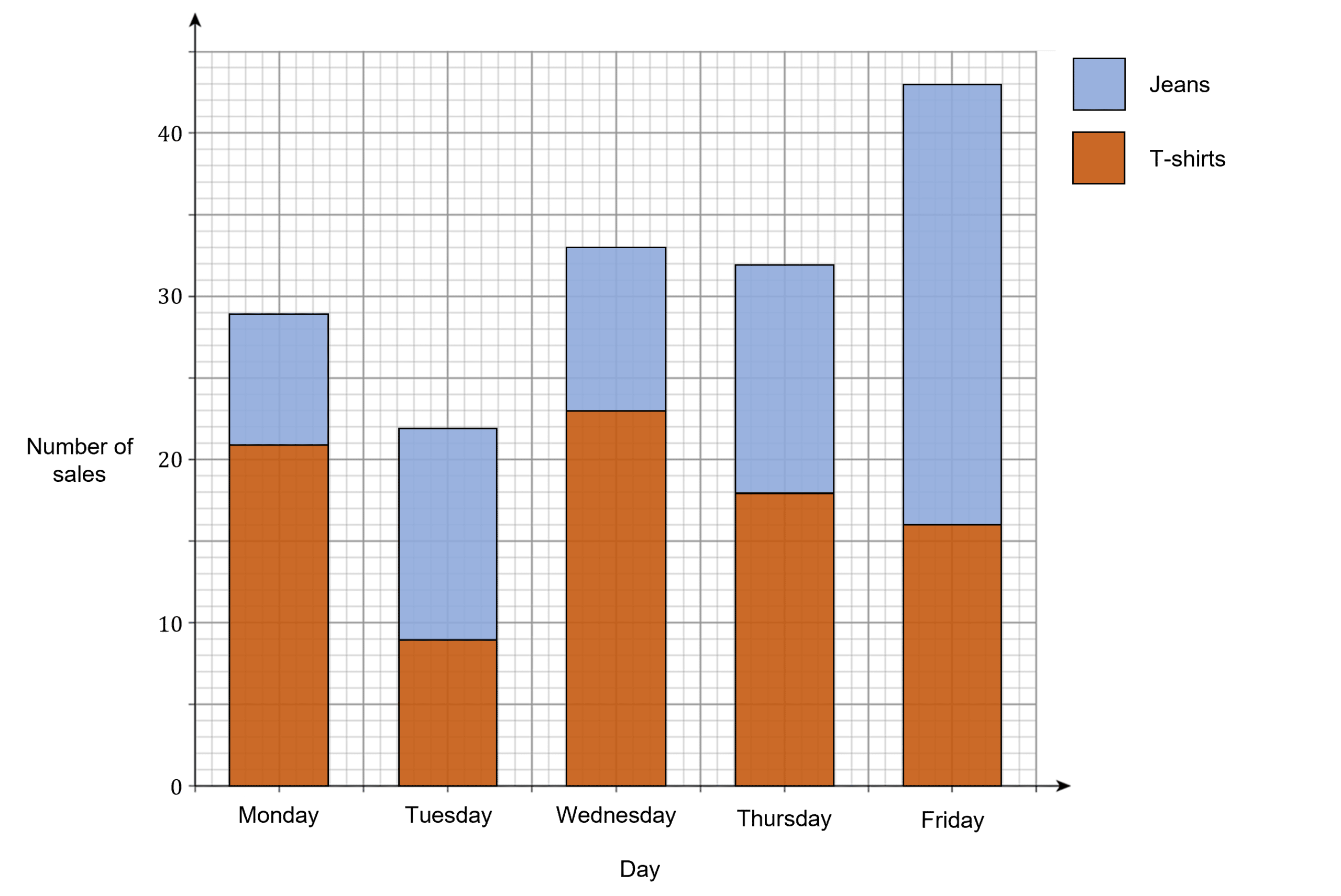Level 1-3GCSEKS3AQAEdexcelOCRWJECCambridge iGCSEEdexcel iGCSE

## Bar Graphs Example Questions

Your bar graph should look like the one shown below. Remember, you must have gaps between the bars and everything (including the axes and the individual bars) should be clearly labelled.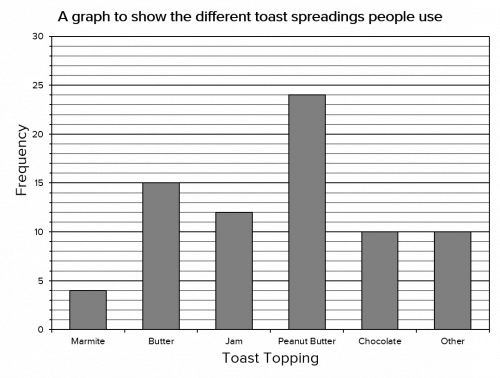Gold Standard Education

a) According to the scale on the $y$-axis, $5$ small squares accounts for $2,000$ book sales, so:

$\text{One small square }=2,000\div 5=400\text{ books}$

So, that means the number of audiobooks sold is $3,000$, the number of hardbacks sold is $5,000$, and the number of paperbacks sold is $12,000$. Therefore the percentage of sales that were audiobooks is:

$\dfrac{3,000}{3,000+5,000+12,000}\times 100=15\%$

b) We’ve already determined the number of hardbacks and paperbacks sold, so the ratio is:

$5,000 : 12,000 = 5 : 12$Gold Standard Education

a)  For this question, we need to take a reading of the two bars showing the $0 – 5$ servings per week category. The number of adults who have between $0$ and $5$ servings per week is a total of $4$. The number of children who have between $0$ and $5$ servings per week is a total of $16$.

Therefore, the number of adults and children combined who have between $0$ and $5$ servings is $4+16 = 20$

b) For this question, we simply need to locate the bar that represents children eating over $20$ servings and the bar that represents children eating between $6 – 10$ servings, and work out the difference between them.

The number of children who eat over $20$ servings of fruit and vegetables is $4$. The number of children who eat between $6$ and $10$ servings of fruit and vegetables is $25$. Therefore, the difference between these two categories is $25-4=21$

c) The mode is the most frequently occurring value.  Since this question is about the adults, we do not need to consider the bars for children.  All we need to do is see which bar is highest for the adults.

The $16 – 20$ bar is the highest with $22$ (the actual number $22$ doesn’t really need to be considered since we can clearly see that the bar contains the most values since it is obviously higher than the other adult bars). Therefore the mode is $16 – 20$ servings.

d)  The most basic trend we can obtain from the given information is that adults eat more portions of fruit and vegetables per week than children.Gold Standard Education

a)  For this question, we need to locate the bar that corresponds to girls and $6-10$ hours and take a reading from the top of the bar. Doing so we find $12$ girls played video games for $6-10$ hours.

b)  We need to be careful with this question as It is asking for less than $16$ hours, so this means we need to consider all the data to the left of the $16-20$ hours bar. The number of boys who played video games for $11-15$ hours was $8$ and the number of boys who played $6-10$ hours was $2$.

Therefore the number of boys who played for less than $16$ hours was $8+2=10\text{ boys}$.

c)  For this question, we are looking for $10$ hours or less for the girls.  This means that we need to look at the first two bars. The number of girls who played for $6-10$ hours was $12$ and the number who played for $0-5$ hours was $8$. Therefore the number of girls who played video games for $10$ hours or less was $12+8=20\text{ girls}$.

This means that $20$ out of the total number of girls played for $10$ hours or less.   However, we don’t know how many girls there were in total, so we will need to add up the totals for each bar. Doing so we find that in total there are $8+12+20+14+8+10+6+2=80\text{ girls}$.

Thus $20$ out of the $80$ girls play video games for $10$ hours or less, which works as $25%$.

d)  To answer this question, we need to work out how many boys there are in each category, the total time spent playing video games for all the boys, and how many boys there are in total.

The total number of boys is $2+8+12+10+18+16+14=80\text{ boys}$

If $2$ boys played for $9$ hours, then this represents $18$ hours of playing time.

If $8$ boys played for $10$ hours, then this represents $80$ hours of playing time.

If $12$ boys played for $11$ hours, then this represents $132$ hours of playing time.

If $10$ boys played for $12$ hours, then this represents $120$ hours of playing time.

If $18$ boys played for $13$ hours, then this represents $234$ hours of playing time.

If $16$ boys played for $14$ hours, then this represents $224$ hours of playing time.

If $14$ boys played for $15$ hours, then this represents $210$ hours of playing time.

Therefore the total number of hours of playing time for the boys combined is $18+80+132+120+234+224+210=1018\text{ hours}$.

If there are $80$ boys who play for a combined total of $1018$ hours, then the mean number of hours can be calculated as follows:

$\dfrac{1018\text{ hours}}{80\text{ boys}} = 13 \text{ hours}$Gold Standard Education

For the vertical $y$-axis, we will be plotting the frequency (the number of boys / girls).  The only thing we need to consider is the scale.  Since the lowest value is $0$ and the highest value is $9$, the $y$-axis needs to start at $0$ and needs to finish at $9$ (although the axis can extend slightly beyond $9$ if you like).

It is probably the $x$-axis that is going to cause more problems.  Since this is a dual bar chart, the boys and girls columns need to be side by side, but there needs to be a gap between each category.  In other words, the bars for boys-that-walk and girls-that-walk touch each other, but the walking and biking categories do not touch since they are completely separate categories.

The widths of the bars should all be the same, as should the gaps between the categories.

Your end result should be similar to the one below: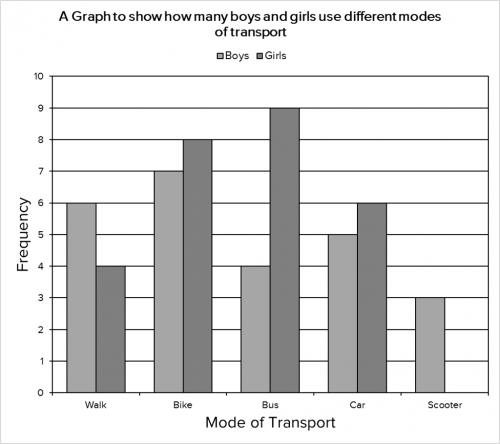Gold Standard Education

From the bar chart we can see that $21$ people prefer the drama genre.

Number of adults who prefer drama $=21-7=14$

Total number of adults $=(28-11)+(32-14)+(21-7)+(11-1)+(37-31)=65$

Percentage of adults who prefer drama $=\dfrac{14}{65}\times 100=21.5\%$ (to one decimal place)Gold Standard Education

## Bar Graphs Worksheet and Example Questions

### (NEW) Bar Graphs Exam Style Questions - MME

Level 1-3GCSENewOfficial MMEGold Standard Education

Level 4-5GCSE

Level 1-3GCSEKS3